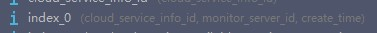### SQLAlchemy 设置多列复合索引

`op.drop_index('index_0', table_name='monitor')``# 云服务器提供商网络质量监控数据class Monitor(db.Model):    __tablename__ = 'monitor'    id = db.Column(db.Integer, primary_key=True)    avg_time = db.Column(db.Float)    create_time = db.Column(db.DateTime(timezone=True), server_default=func.now(), index=True)    info_id = db.Column(db.Integer, db.ForeignKey('service_infos.id'))    server_id = db.Column(db.Integer, db.ForeignKey('server_list.id'),                                  doc='那个监控服务器发送过来的数据')    __table_args__ = (Index('index_0', info_id, server_id, create_time),)`